StatLect

# Dummy variable

In regression analysis, a dummy variable is a regressor that can take only two values: either 1 or 0.

Dummy variables are typically used to encode categorical features.## Example

Suppose that we want to analyze how personal income is affected by:

• years of work experience;

To do so, we can specify a linear regression model as follows: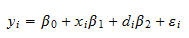where:

• the subscriptdenotes an individual in our sample;

• the dependent variableis a measure of income;

•is the number of years of work experience;

•is a dummy variable, equal to 1 if the individual has a higher degree or other postgraduate qualification and 0 otherwise;

•is the error of the regression;

•is the intercept of the regression;

•andare the regression coefficients of the two variables.

## Interpretation

In the previous example,is the regression coefficient of the dummy variable. It measures by how much postgraduate education raises income on average.

In general, the regression coefficient on a dummy variable gives us the average increase inobserved when the dummy is equal to 1 (with respect to the base case in which the dummy is equal to 0).

## Matrix form

Let us continue with the previous example, to see how a dummy variable looks like when the data is gathered in a matrix or table.

Suppose that our sample is as follows.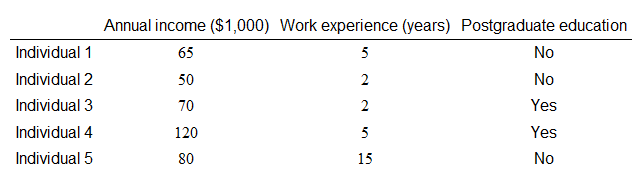After encoding the categorical variable with a dummy, the vectorof dependent variables and the matrix of regressors(so-called design matrix) will be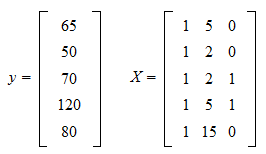Note that the first column contains all 1s because we have included an intercept in the regression.

## Collinearity

We might be tempted to include two dummies in our regression:

1. a first dummy that is equal to 1 if the individual has a higher degree and 0 otherwise;

2. a second dummy that is equal to 1 if the individual does not have a higher degree and 0 otherwise.

In our previous example, the design matrix would becomeThe problem with this double encoding is that our regressors become perfectly multicollinear, that is, one of the columns ofis equal to a linear combination of the other columns.

In our example, we have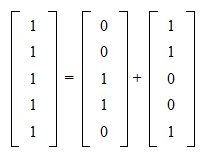With perfect multicollinearity, the design matrixbecomes singular, which implies that we cannot estimate the regression coefficients with Ordinary Least Squares (OLS).

In fact, we can compute the OLS estimator only ifis full-rank. We can still compute estimators such as the Ridge, which do not requireto be full-rank.

## More than two categories

Thus, when we have an intercept in the regression model and we want to avoid perfect multicollinearity, we create only one dummy to encode a categorical variable that has two categories.

Similarly, we createdummies to encode a categorical variable that hascategories.

The category that is not encoded into a dummy becomes the base category.

## Example with three categories

Let us make an example with three categories.

Suppose that our sample is similar to the previous one, but individuals have been divided into three groups (H, M and L) based on their education.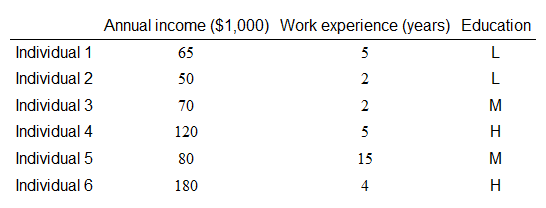If we choose L as the base category, then we create two dummies:

1. the first dummyis 1 if the category is M and 0 otherwise;

2. the second dummyis 1 if the category is H and 0 otherwise.

The vectorof dependent variables and the matrix of regressors(so-called design matrix) are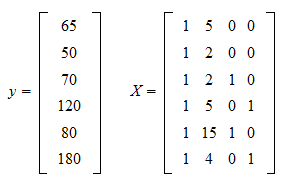The regression equation is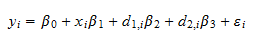The regression coefficients of the two dummies are interpreted as follows:

•is the average increase inobserved when the category is M (with respect to the base case in which the category is L);

•is the average increase inobserved when the category is H (with respect to the base case in which the category is L).

## Dropping the intercept

An alternative to encoding onlyof thecategories as dummies is to drop the intercept and encode all thecategories.

With the data in the previous example, we could have done: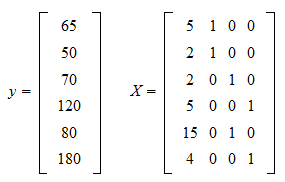where we have encoded L, M and H into three dummies,and.

The regression equation is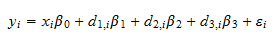The interpretation of regression coefficients changes:

•is the intercept of the regression when the category is L (i.e., the average income of an L individual with no work experience);

•is the intercept when the category is M;

•is the intercept for H individuals.

## One-hot encoding

In machine learning, the practice of encodingcategories intodummies is often called one-hot encoding.